## Introduction

The formation of minibands is a common moiré superlattice (mSL) effect1,2,3,4,5,6,7,8,9,10,11,12,13,14, often related to a rigid rotation of one 2D crystal against the other. In general, the approximation of a rigid interlayer twist is valid for lattice-mismatched crystals or larger twist angles, where a short mSL period prohibits the formation of energetically preferential stacking domains of the two crystals. In contrast, for marginally (small-angle) twisted bilayers of crystals with very close lattice constants, the long period of the mSL offers sufficient space for creating preferential stacking areas. That is, the energy gain due to better adhesion can surmount the cost of intralayer strain in each of the constituent crystals. The reconstruction of small-angle twisted bilayers into a network of domains15 [2H for antiparallel (AP) and 3R for parallel-oriented (P) bilayers] has been observed in various bilayers of transition metal dichalcogenides (TMDs)16,17,18,19,20. The observed16,17,18,19,20 and theoretically modelled15,21,22 structures feature hexagonal (for AP) and triangular (for P) NoDW with nodes hosting few nanometer areas of “chalcogen-on-chalcogen” stacking (XtXb), which are hot spots of the intralayer strain.

Below we study the effects produced by these hot spots of strain in marginally twisted same-chalcogen heterobilayers MoX2/WX2. While domains/NoDW form in both homo- and heterobilayers, the in-plane intralayer deformations, u(r), in those two systems are qualitatively different. In homobilayers the formation of preferential stacking domains is brought about by twisting locally the crystals toward each other. As a result, the deformations at the domain walls are predominantly shear in character, that is, with $${{{\rm{div}}}}\,{{{\boldsymbol{u}}}}\equiv {u}_{ii}\to 0$$, where $${u}_{ij}\equiv \frac{1}{2}({\partial }_{i}{u}_{j}+{\partial }_{j}{u}_{i})$$ is a 2D strain tensor, and uii is its trace. In perfectly aligned (θ = 0°) heterobilayers, lattice mismatch (δ ≈ 0.2% for MoS2/WS2 and δ ≈ 0.4% for MoSe2/WSe2) requires an adjustment of the MoX2 ($${u}_{ii}^{{{{\rm{Mo}}}}}\approx -\delta$$ compression) and WX2 ($${u}_{ii}^{{{{\rm{W}}}}}\approx \delta$$ expansion) lattices toward each other inside the large area domains. This inflicts a few percent of hydrostatic compression of WX2 and expansion of MoX2 in XtXb areas (NoDW nodes), quantified in Figs. 1 and 2, which, as we demonstrate below, create deep confinement potentials for charge carriers and interlayer excitons (iXs). Theoretical modelling23,24,25,26,27 of localized iXs in WX2/MoX2 bilayer has been attempted earlier, however without taking into account strong lattice relaxation effects (which is applicable to structures with larger misalignment angles, θ > 2°). This led to the underestimation of the depth of the size of the band edge variation for electrons and holes, as compared to what we find in this Letter, and with different positioning of band edge extrema across moiré supercell. In this work we focus on small-angle twisted WX2/MoX2 bilayers where lattice relaxation plays the critical role on trapping charge carriers, in particular due to a substantial hydrostatic strain component at NoDW nodes. Up to now, no optical studies have been reported on such small-angle (θ ≤ 1°) bilayers, despite that the structural features of NoDW have been demonstrated using transmission electron microscopy16,17 and several spectroscopic studies28,29,30,31 have been performed on bilayers with larger misalignment angles, where lattice reconstruction does not play such a dominant role as discussed below.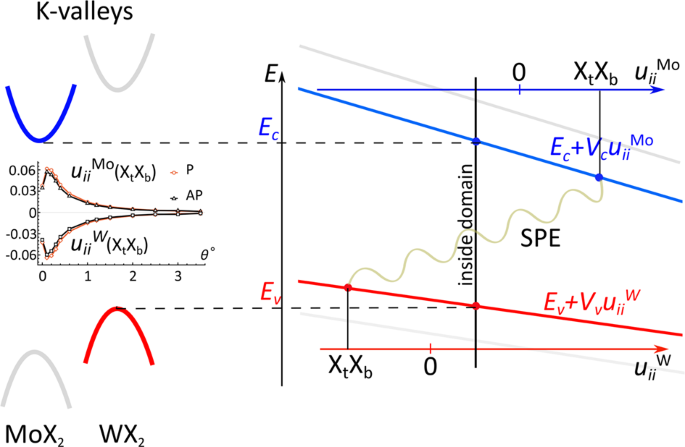Fig. 1: Variation of K-valley conduction/valence, Ec/v, band edges in MoX2 (blue) and WX2 (red) monolayers under hydrostatic strain with alignment of the bands corresponding to lattice-matched WX2/MoX2 heterobilayers.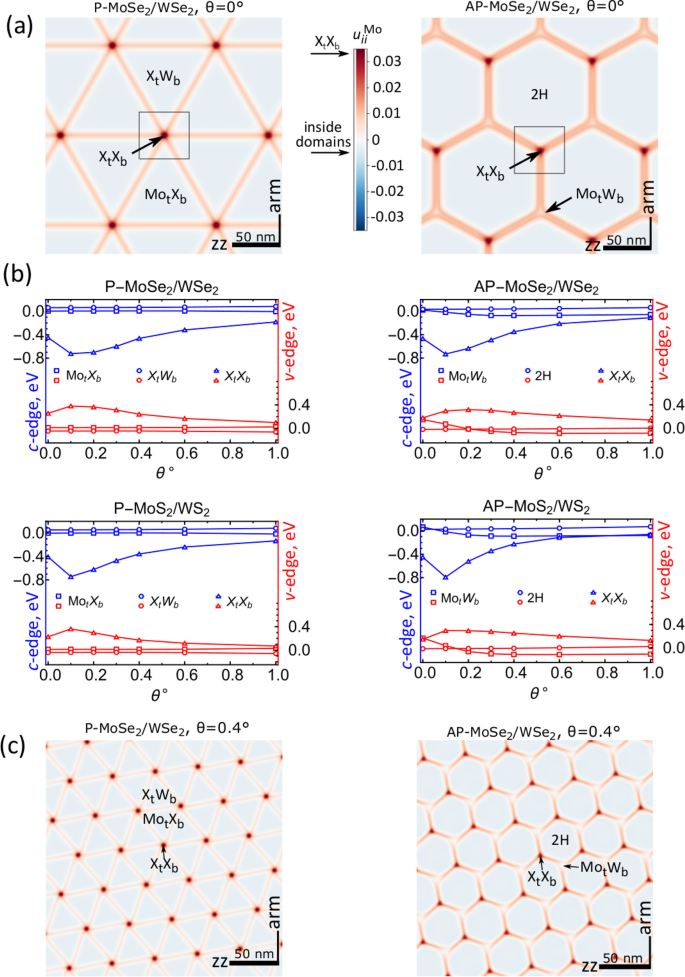Fig. 2: Lattice reconstruction and local conduction and valence band edge modulations at K-valleys in twisted MoX2/WX2 bilayers.

## Results and discussion

### Modulation of band edges by strain and charge transfer

Here, we single out the hydrostatic strain component because of the critical role it plays in determining the K-valley energies in MoX2/WX2 crystals. Several earlier experimental and density functional theory (DFT) studies32,33,34 have agreed that conduction and valence band edges in TMD monolayers are strongly shifted by hydrostatic strain, but without much sensitivity to shear deformations. This trend is illustrated in Fig. 1. The corresponding shifts of conduction/valence band edges in MoX2/WX2 determine the energy of the interlayer exciton (iX). Inside domains formed by lattice reconstruction, hydrostatic strains compensate lattice mismatch between the layers: this slightly increases the band gap as compared to rigidly twisted bilayers without strain (Fig. 1). Compensating small deformations inside large domain areas, $${u}_{ii}^{{{{\rm{Mo,W}}}}}$$ in XtXb nodes have the opposite signs and much larger magnitudes as compare to $${u}_{ii}^{{{{\rm{Mo,W}}}}}$$ inside domains. This strongly decreases layer-indirect band gap and determines deep confinement potentials for both electrons and holes, leading to the appearance of SPEs.

The intralayer strain ($${u}_{ii}^{{{{\rm{Mo/W}}}}}$$) maps in Fig. 2 were computed using a multiscale modelling approach15, tested in the detailed comparison with the STEM microscopy data16. This approach starts with the computation of stacking-dependent MoX2/WX2 adhesion energy, $${{{\mathcal{W}}}}$$, followed by the parametrization of interpolation formulae15 for its dependence on the interlayer lateral offset, r0. For both P and AP bilayers, energetically favorable stackings are those with the largest lateral separation between chalcogens. These stackings are MotXb and XtWb for P-orientation and 2H for AP-orientation. Note that XtXb stacking is unfavorable energetically, and its interlayer distance swells15 by up to ≈0.5 Å. By combining interpolation formulae for $${{{\mathcal{W}}}}({{{{\boldsymbol{r}}}}}_{0})$$15 where we use local lateral offset,

$${{{{\boldsymbol{r}}}}}_{0}({{{\boldsymbol{r}}}})=\delta \cdot {{{\boldsymbol{r}}}}+\theta \hat{z}\times {{{\boldsymbol{r}}}}+{{{{\boldsymbol{u}}}}}^{{{{\rm{Mo}}}}}-{{{{\boldsymbol{u}}}}}^{{{{\rm{W}}}}},$$
(1)

with elasticity theory and minimizing total energy of the bilayer across its mSL (which period, $$\ell \approx a/\sqrt{{\theta }^{2}+{\delta }^{2}}$$, is fixed by the twist angle and lattice mismatch between the crystals), we compute the deformation fields.

In Fig. 2a, c, we present $${u}_{ii}^{{{{\rm{Mo}}}}}$$ maps for MoSe2/WSe2 bilayers with θ = 0° and θ = 0.4°, where the preferential stacking domains (triangular/hexagonal for P/AP-bilayer) are separated by networks of dislocation-like domain walls with XtXb stacking at the NoDW nodes15. Note that for small twist angles the hydrostatic component of strain persists, now combined with shear deformations (similar to those in twisted homobilayers).

To incorporate strain into the shifts of conduction/valence band edges, δεc/v, we performed DFT modelling of the TMD band structures using Quantum ESPRESSO35 (see Methods). The computed variations of all band edges can be described as ≈ Vv/cuii, with $${V}_{c}^{{{{\rm{Mo{S}}}_{2}}}}=-12.45$$ eV, $${V}_{v}^{{{{\rm{W{S}}}_{2}}}}=-5.94$$ eV, $${V}_{c}^{{{{\rm{MoS{e}}}_{2}}}}=-11.57$$ eV, $${V}_{v}^{{{{\rm{WS{e}}}_{2}}}}=-5.76$$ eV, which are quoted for the relevant bilayer bands. Using these values, we compute,

$$\delta {\varepsilon }_{v/c}({{{\boldsymbol{r}}}})={V}_{v/c}{u}_{ii}^{{{{\rm{W/Mo}}}}}({{{\boldsymbol{r}}}})-e{\phi }_{{{{\rm{piezo}}}}}^{{{{\rm{W/Mo}}}}}({{{\boldsymbol{r}}}})\pm \frac{1}{2}\Delta ({{{\boldsymbol{r}}}}),$$
(2)

taking into account strain-dependent piezoelectric potential15, $$-e{\phi }_{{{{\rm{piezo}}}}}^{{{{\rm{W/Mo}}}}}({{{\boldsymbol{r}}}})$$, and offset-dependent potential drop, Δ(r), due to interlayer charge transfer (for details see Supplementary Section 1). The first term in Eq. (2) represents the effect of hydrostatic component of the intralayer strain which was missed in the previous analysis of the same systems36. The twist-angle dependences of the computed band edge energies δεc/v for three selected stacking areas (MotXb, XtWb, XtXb for P and 2H, MotWb, XtXb for AP) are plotted in Fig. 2(b). These figures suggest that XtXb regions are potential wells for electrons and holes and these wells are the deepest for θ ≈ δ. Based on that we describe the NoDW nodes as trigonally warped quantum dots (QDs), with band edge profiles exemplified in Fig. 3. These QDs are sufficiently deep to accommodate at least two size-quantized states for electrons/holes which retain their distinct s (Lz = 0) and p (Lz = ± 1) characteristics due to the $${\hat{C}}_{3}$$-symmetry of the dots.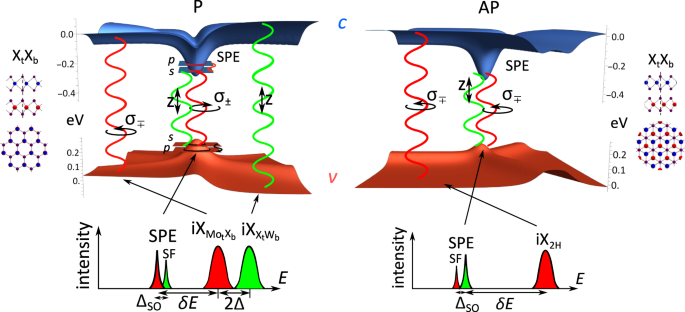Fig. 3: Self-organized quantum dots and spectral features of SPE and iX.

Note that QD formation in marginally twisted structures qualitatively differs from band energy profiles in stronger misaligned P-bilayers with θ ≥ 2°, where the band edges at K-valley shift into MotXb stacking areas, see Fig. 4. This crossover agrees with the findings of Refs. 23,37. In addition, for MoS2/WS2 with θ ≈ 1.8° and MoSe2/WSe2 with θ ≈ 2.4° the energy profile for interlayer interband exciton resembles an antidot superlattice more than an array of QDs. This contrasts with the persistence up to θ ~ 3.5° of shallow QD arrays for both electrons and holes based at XtXb areas in AP-bilayers.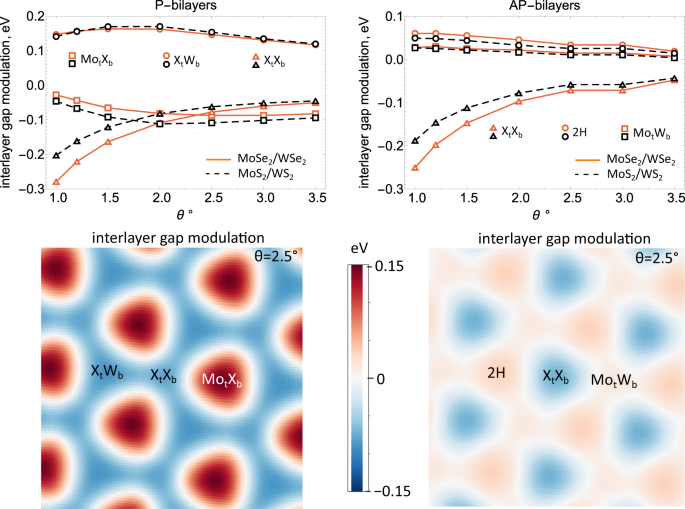Fig. 4: (Top) Twist-angle-dependences of interlayer band gap at K-valleys at high symmetry stacking areas of moiré supercells of P- and AP-MoX2/WX2 bilayers with 1 ° ≤ θ ≤ 3.5°.

### Spectral characteristics of self-organized QDs

Spectral features of the interlayer interband emissions of self-organized QDs of marginally twisted bilayers are sketched on the bottom insets in Fig. 3. Energy separation, δE, between the QD transition and the iX inside the domains was computed as,

$$\begin{array}{rcl}\delta E&=&{\varepsilon }_{e}^{(s)}-{\varepsilon }_{h}^{(s)}-{E}_{{{{\rm{iX}}}}}\\ &&-\int \int {d}^{2}{{{\boldsymbol{r}}}}{d}^{2}{{{\boldsymbol{r}}}}^{\prime} {\left|{\psi }_{e}^{(s)}({{{\boldsymbol{r}}}})\right|}^{2}{\left|{\psi }_{h}^{(s)}({{{\boldsymbol{r}}}}^{\prime} )\right|}^{2}{V}_{eh}({{{\boldsymbol{r}}}}-{{{\boldsymbol{r}}}}^{\prime} ).\end{array}$$
(3)

Here, EiX is the iX energy and $${\varepsilon }_{e/h}^{(s)}$$ are the energies of the electron/hole s-states $${\psi }_{e/h}^{(s)}$$ inside quantum well. We also take into account the interlayer e-h attraction, Veh, screened by the in-plane susceptibility of TMDs and hBN environment38 (see details in Supplementary Sections 2 and 3). The computed dependences of δE(θ) for MoX2/WX2 bilayers (X = Se,S) are shown in Fig. 5. We find that the QD line can be tuned across a 0.8–1.2 eV spectral interval (telecom range) for 0.3° ≤ θ ≤ 1° in MoSe2/WSe2 and for 0° ≤ θ ≤ 0.5° in MoS2/WS2. In Fig. 5 the computed data for electrons in AP-MoS2/WS2 are terminated at θ = 0.6°, because for a larger misalignment the K-valley conduction band profile starts resembling an antidot lattice with maxima at the 2H domains (see Fig. 2(b)).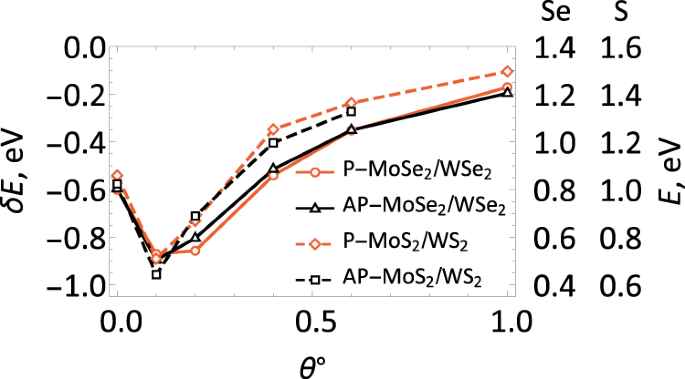Fig. 5: Energies of SPEs in P/AP-WX2/MoX2 bilayers shown as a shift from the lower iX energy inside the domains (left-side axis common for all bilayers) and as an absolute value (right-side axis specified separately for selenides and sulfides).

### Polarization and spin selection rules

Additional information, displayed in Fig. 3 and gathered in Table 1, concerns the polarizations of SPEs and iXs inside domains and fine structure related to a ΔSO-splitting39,40,41,42,43 between the spin-flip and spin-conserving interband transitions inside the QDs. We note that, in each of the two ± K-valleys, the hole spin at the band edge is determined by the spin-valley locking in WX2, whereas conduction band in MoX2 is characterized by spin-orbit splitting ΔSO. Also, the iX emission from the inner part of domains, shown in Fig. 3, differs for P and AP bilayers. For AP-bilayers we expect a single line of circularly polarized iX emission. For P-bilayers iX energies and polarizations are different for MotXb and XtWb domains, with the energy splitting determined by the interlayer charge transfer36,44,45 and circular (in MotXb) vs linear (in XtWb) polarization, established in Ref. 46. In Table 1 we underline the most prominent SPE transition which happens to be related to the spin-conserving recombination in self-organized QDs in P-bilayers with approximately one hundred times weaker intensity than that of intralayer A-exciton (AX) in MoX2 layer determined by the ratio of corresponding interband matrix elements. To mention, DFT modelling suggests that lattice matching inside domains promotes direct-to-indirect band gap crossover for electrons toward Q-valley and most importantly for holes toward Γ-valley47 (see also Supplementary Section 6).

### Intralayer transitions in self-organized QDs

In addition, intraband sp optical transitions for electrons/holes trapped in QDs give rise to infrared (IR) features with energies shown in Fig. 6(a) for twist angles 0° ≤ θ ≤ 1°. In AP-bilayers, XtXb NoDW nodes also feature spikes of pseudomagnetic field B*15 characteristic of multivalley semiconductors with a strongly inhomogeneous strain48,49,50. These pseudomagnetic fields have opposite signs for electrons in ±K-valleys, splitting (by Δν = 5μBB*) the QD sp transitions into circularly polarized doublets, as sketched in Fig. 6(a). Such an IR transition can be used to manipulate the state of the SPE, by exciting either electron or hole into their respective QD p-states.

The intralayer band gap variation, due to the hydrostatic strain at XtXb nodes reduces/increases the energy of the intralayer AX in MoX2/WX2. In Fig. 6(b) we show that this results in a~100 meV potential well for AX in MoX2 exactly over the self-organized QD position. The red-shift of the MoX2 AX confined in such a well can be used for selective population of the QD states, upon the relaxation of photoexcited hole in MoX2 layer into its bound state in the QD in WX2.

### Discussion

Overall, hot spots of hydrostatic strain at the nodes of domain wall network, generated by the lattice reconstruction in marginally twisted MoX2/WX2 bilayers, form a nanoscale array of QDs for electrons and holes, which may be operated as single-photon emitters. Based on the presented analysis, we propose that the SPE spectrum can be tuned by the choice of the twist angle over a broad range (including telecom for MoSe2/WSe2 bilayers), and the electron/hole state in these QDs can be manipulated via intra-band sp transitions using THz radiation. The data on the optical oscillator strength of the interlayer interband transitions in such QDs, Table 1, suggest that the brightest would be SPEs in marginally twisted biayers with parallel orientation of MoX2 and WX2 unit cells. Ratio of intra- and inter-layer interband velocity matrix elements also suggest that the recombination rate of QD-localized excitons is about ~1% of the recombination rate of the intralayer A-exciton in MoX2; as the latter was found in Refs. 51,52,53,54 to be ~1/300 − 1/200 fs−1, this would set a 100 MHz possible repetition rate for SPEs in these self-organized QDs. Note that areal density of these SPEs is ~1011 cm−2, which is 100 times higher than the density of quantum emitters in patterned TMD monolayers55,56 and that a red-shift of the A-exciton in MoX2, due to the same hot spots of strain, would enable selective population of the QD states for the optical pumping of the self-organized SPEs.

Finally, we note that in real samples there is usually inhomogeneity of domain structure caused by smooth strain introduced during sample transfer process. Such inhomogeneity spread a spectral range of SPEs available on a single wafer, which can be used to produce a wealth of SPE devices operating in complementary spectral intervals provided by different parts of a single large-area WX2/MoX2 bilayer.

## Methods

### Computation of hydrostatic strain effect

To quantify effect of hydrostatic strain on the band edges of TMD monolayes we considered biaxial strain in the range of ±2%, fully relaxing atomic positions in the monolayer with Vanderbilt PBE GBRV ultrasoft pseudopotentials57, a wavefunction cut-off of Ecut = 50 Ry, and a 20 × 20 × 1 k-point grid, sampled according to the Monkhorst-Pack algorithm58. Spin-orbit coupling was included by a norm-conserving fully-relativistic pslibrary PBE PAW pseudopotentials(We used the relevant relativistic pseudopotentials from http://www.quantum-espresso.org.) with Ecut = 80 Ry.